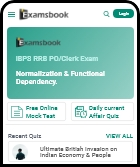913 0

## Q: What is the product of a number and its reciprocal?

• 1
0
• 2
1
• 3
-ve of the number
• 4
the number itself
• Workspace

## Answer : 2. "1" Explanation :Answer: B) 1 Explanation: The product of a number and its reciprocal is always equals to 1.   For Example : Let the number be 4. Now, its reciprocal is 1/4 Hence, required product = 4 x 1/4 = 1.   Now, take the number as -15. Then its reciprocal is -1/15 Required product = -15 x -1/15 = 1.   Hence, the product of a number and its reciprocal is 1.

### Are you sure